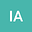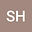Lucas and Fibonacci polynomials based approach for the study of one- and two-dimensional Burger and heat-type equations
••• Ihteram Ali,
• Sirajul Haq
Ihteram Ali
Ghulam Ishaq Khan Institute of Engineering Sciences and Technology
Author ProfileSirajul Haq
Ghulam Ishaq Khan Institute of Engineering Sciences and Technology
Author Profile#### Peer review status:UNDER REVIEW

27 Apr 2020Submitted to Mathematical Methods in the Applied Sciences
01 May 2020Assigned to Editor
01 May 2020Submission Checks Completed
01 May 2020Reviewer(s) Assigned

## Abstract

In this work a numerical technique, combination of Lucas and Fibonacci polynomials, is proposed for the solution of one- and two-dimensional nonlinear heat type equations. In first round, for discretization, finite difference has been used for time and Crank Nicolson scheme for spatial part. In second round, the unknown functions have been approximated by Lucas polynomial while their derivatives by Fibonacci polynomials. With the help of these approximations, the nonlinear partial differential equation transforms to a system of algebraic equations which can be solved easily. Convergence of the method has been investigated numerically. Performance of the method has been studied by taking one- and two-dimensional heat and burger equations. Efficiency of the technique has been investigated in terms of root mean square (RMS), L2 and L_infnty norms. The obtained results are then compared with those available in the literature.